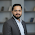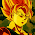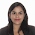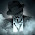» » Program to print prime numbers in java

# Program to print prime numbers in java

1. package com.instaceofjava;
3.
4. public static void main(String[] args) {
5.
6. int num=50;
7. int count=0;
8.
9. for(int i=2;i<=num;i++){
10.
11. count=0;
12.
13. for(int j=2;j<=i/2;j++){
14.
15. if(i%j==0){
16. count++;
17. break;
18. }
19.
20. }
21.
22. if(count==0){
23.
24. System.out.println(i);
25.
26. }
27.
28. }
29.
30. }
31.
32. }

Output:
1. 2
2. 3
3. 5
4. 7
5. 11
6. 13
7. 17
8. 19
9. 23
10. 29
11. 31
12. 37
13. 41
14. 43
15. 47

Java programming interview questions

#### 7 comments for Program to print prime numbers in java

1.how this count functions in this program?

2.public class Print_prime {

public void findPrime (int start_number, int last_number){
System.out.println("prime number between "+start_number+" and "+last_number
+" are");
for (int i = start_number; i < last_number+1; i++) {
if(i%2 == 0){

System.out.println(i);
}
}
}

public static void main(String[] args) {
Print_prime pp = new Print_prime();
pp.findPrime(10, 101);
}

}

1.your program is to print even numbers, not prime

2.Yes, the above program is not correct. To print prime numbers, the logic mentioned here is not correct. Please see correct logic to print all prime numbers till the input upperbase number

import java.util.Scanner;

/**
* @param args
*/
public static void main(String[] args) {
// TODO Auto-generated method stub

}

int upperBase;
int num;
System.out.println("Enter the upper base number:");
Scanner input = new Scanner(System.in);
upperBase = input.nextInt();
input.close();

System.out.println("\nPrime numbers between 0 and "+upperBase+" are:\t");

for(int i=2; i<=upperBase;i++){

int count=0;

for(num=i;num>1;num--){

if(i%num==0){
count++;

}
}

if(count==1){
System.out.println(i);
}

}

}
}

3.4.i want to print above prime numbers in desending order.but not the way replaceing values of for loop value.values will be same.

5.Good work sir, Thanks for the proper explanation about JAVA . I found one of the good resource related JAVA and OOPS concepts. It is providing in-depth knowledge on JAVA and OOPS. which I am sharing a link with you where you can get more clear on JAVA and OOPS. To know more Just have a look at this link

Java Tutorial
Class and object
Inheritance
Polymorphism
Abstraction
Encapsulation
,,